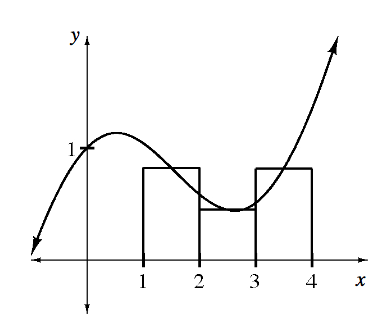### Home > APCALC > Chapter 6 > Lesson 6.1.4 > Problem6-51

6-51.Midpoint rectangles have their height defined at the midpoint of the interval. For the function

$f(x)=\frac { 1 } { 2 }x + \cos(x)$

graphed at right, you previously found the heights to be $f(1.5) ≈ 0.821,$$f(2.5) ≈ 0.449,$ and $f(3.5) ≈ 0.814$.

1. Approximate the area under the curve for $1 ≤ x ≤ 4$ using midpoint rectangles.

There are only three rectangles.
No need to use sigma notation.

Just find the area of each rectangle and add. Be sure to organize your work in a way that others will understand your process.

2. Calculate the exact area and compare it with your approximation.

Find the exact area using a definite integral.

$\int_1^4\frac{1}{2}x+\cos(x)=$